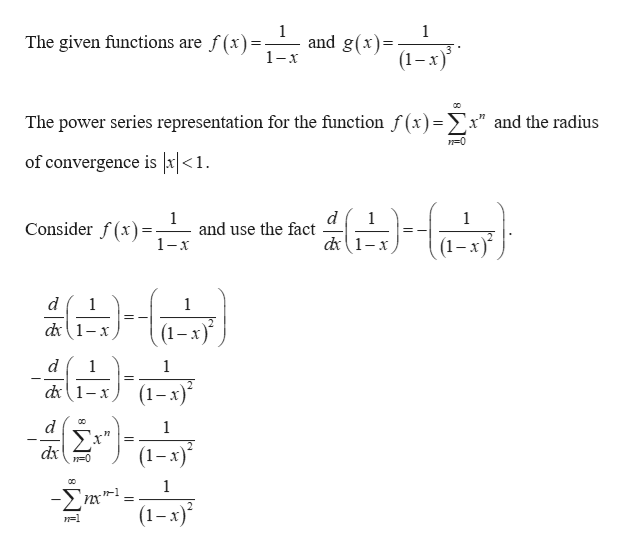# Find the power series representation for g(x) centered at 0 by differentiating or integrating the power series for f(x). Give the interval of convergence for the resulting series.f(x) = [1/(1-x)]   ;   g(x) = [1/(1-x)3]

Question
52 views

Find the power series representation for g(x) centered at 0 by differentiating or integrating the power series for f(x). Give the interval of convergence for the resulting series.

f(x) = [1/(1-x)]   ;   g(x) = [1/(1-x)3]

check_circle

Step 1help_outlineImage Transcriptionclose1 The given functions are f(x 1 and g(x) 1-x (1-х) The power series representation for the function f (x) =x" and the radius of convergence is x|<1. d 1 and use the fact 1 1 Consider f(x)=- d1-x 1-x d 1 1 d1-x d 1 1 d1-x - 1 Σ" (1-x) dx 1 -nx' = (1-x)* 1=1 fullscreen

### Want to see the full answer?

See Solution

#### Want to see this answer and more?

Solutions are written by subject experts who are available 24/7. Questions are typically answered within 1 hour.*

See Solution
*Response times may vary by subject and question.
Tagged in

### Other